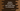# Python dictionary example to find keys with the same value# Introduction :

A python dictionary is a collection of key-value pairs. All keys in a python dictionary should be different.No two keys can have the same name. But for different keys, we can have the same ’value’. This tutorial is to show you how we can find all keys in a python dictionary with the same value.

We will create one program that will :

1. Create one dictionary by taking the inputs from the user.
2. The user will enter one value to find in the dictionary.
3. It will find_ if more than two keys have the same value_ entered by the user.
4. The program will also print out the keys for that value if found.

## Solve it by using a loop :

This is the simplest option to look for anything in a dictionary. At first, the program will get the full list of key-value pairs from the dictionary and then using one_ for loop_, it will compare each pair one by one.

We will take all inputs from the user, i.e. the user will enter the key-value pairs for the dictionary and also the value.

### Python program :

Before going into details, let me show you the python program :

``````#1
given_dict = {}
result_set = set()

#2
total_values = int(
input("Enter total no of key-value pairs of the dictionary : "))

#3
for i in range(total_values):
#4
key_value_str = input(
"Enter key and value for index {} separated by ',' : ".format(i))
#5
key_value = key_value_str.split(',')

#6
given_dict[key_value] = key_value

#7
value_to_find = input("Enter value to find in the dictionary : ")

#8
dict_item_list = given_dict.items()

#9
for item in dict_item_list:
if item == value_to_find:
#10

#11
print("Following are the keys found for value '{}' : ".format(value_to_find))

for item in result_set:
print(item)``````

(The source code is also shared on Github here):

### Explanation :

The commented numbers in the above program denote the step numbers below :

1. givendict_ is an empty dictionary. We will take the inputs from the user and insert them to this dictionary.resultset_ is an empty set for storing the final keys.
2. Ask the user to enter the total number of key-value pairs for the dictionary. Read and store it in totalvalues_ variable.
3. Run one for loop for totalvalues_ time.
4. Ask the user to enter key and value for the current index separated by a comma and store it in keyvaluestr variable. For example, if the user will enter one,1, we will store ‘one,1’ string in keyvaluestr.
5. Split the variable keyvaluestr by_ ,_ . Store the result in keyvalue_ variable. If the string was “one,1”, after splitting, keyvalue_ will hold_ “one”_ in its 0th index and “1” in its 1st index position.
6. Add the key-value pairs to the dictionary. Remember that the key is stored in the 0th index and the value is stored in the first index.
7. Ask the user to enter the value to find in the dictionary.Store it in valuetofind variable.
8. Using_ items()_ function, get the list of all key-value pairs of the dictionary. Store the list in valuetofind variable.
9. Now, iterate all items in the list one by one using a_ ‘for loop’._
10. For each item in the list, check if any value is equal to the user input value valuetofind. If yes, add the key for that value to the final result set.
11. Finally, using one for loop, print out the list of keys for the user input value.

### Conclusion :

As you have seen that using one loop, we can easily find out all keys for a specific value in python. You can also modify the above program to check for multiple values instead of just one. Try to run the above program using python 3 and drop one comment below if you have any queries.